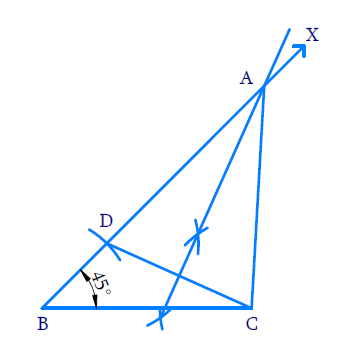# Ex.11.2 Q2 Constructions Solution - NCERT Maths Class 9

Go back to  'Ex.11.2'

## Question

Construct a triangle $$ABC$$ in which $${BC}=8\;\mathrm{cm}, \angle B=\;45^{\circ}$$ and $$AB-AC=3.5\;\rm{cm}.$$

Video Solution
Constructions
Ex 11.2 | Question 2

## Text Solution

Steps of Construction:(i) Draw base $$BC = 8\;\rm{cm}$$ and at point $$B$$ make an angle of using $$45^{\circ}$$ a protractor.

(ii) With $$B$$ as centre and radius $$BD = 3.5\;\rm{cm}$$, draw an arc to intersect ray $$BX$$ at $$D$$.

(iii) Join $$DC$$

(iv) With $$D$$ and $$C$$ as the centre and radius greater than half of $$DC$$. Draw arcs above and below the line to intersect ray $$BX$$ at $$A$$.

(v) Join $$AC$$.$$ABC$$ is the required triangle.

Learn from the best math teachers and top your exams

• Live one on one classroom and doubt clearing
• Practice worksheets in and after class for conceptual clarity
• Personalized curriculum to keep up with school